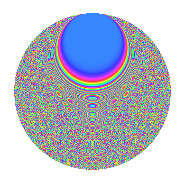# Properties

 Label 1575.2.edLevel 1575 Weight 2 Character orbit ed Rep. character $$\chi_{1575}(53,\cdot)$$ Character field $$\Q(\zeta_{60})$$ Dimension 1280 Sturm bound 480

# Related objects

## Defining parameters

 Level: $$N$$ = $$1575 = 3^{2} \cdot 5^{2} \cdot 7$$ Weight: $$k$$ = $$2$$ Character orbit: $$[\chi]$$ = 1575.ed (of order $$60$$ and degree $$16$$) Character conductor: $$\operatorname{cond}(\chi)$$ = $$525$$ Character field: $$\Q(\zeta_{60})$$ Sturm bound: $$480$$

## Dimensions

The following table gives the dimensions of various subspaces of $$M_{2}(1575, [\chi])$$.

Total New Old
Modular forms 3968 1280 2688
Cusp forms 3712 1280 2432
Eisenstein series 256 0 256

## Trace form

 $$1280q - 8q^{7} + O(q^{10})$$ $$1280q - 8q^{7} - 8q^{10} - 160q^{16} - 112q^{22} + 16q^{25} + 32q^{28} + 16q^{37} + 40q^{40} + 16q^{43} + 80q^{52} + 32q^{55} + 88q^{58} + 32q^{67} - 48q^{70} + 88q^{73} + 216q^{82} - 16q^{85} + 120q^{88} + 352q^{97} + O(q^{100})$$

## Decomposition of $$S_{2}^{\mathrm{new}}(1575, [\chi])$$ into newform subspaces

The newforms in this space have not yet been added to the LMFDB.

## Decomposition of $$S_{2}^{\mathrm{old}}(1575, [\chi])$$ into lower level spaces

$$S_{2}^{\mathrm{old}}(1575, [\chi]) \cong$$ $$S_{2}^{\mathrm{new}}(525, [\chi])$$$$^{\oplus 2}$$

## Hecke Characteristic Polynomials

There are no characteristic polynomials of Hecke operators in the database